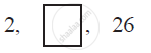# In the following APs, find the missing terms in the boxes - Mathematics

In the following APs, find the missing terms in the boxes :#### Solution

For this A.P.,

a = 2

a3 = 26

We know that, an = a + (n − 1) d

a3 = 2 + (3 − 1) d

26 = 2 + 2d

24 = 2d

d = 12

a2 = 2 + (2 − 1) 12

= 14

Therefore, 14 is the missing term

Concept: nth Term of an AP
Is there an error in this question or solution?
Chapter 5: Arithmetic Progressions - Exercise 5.2 [Page 106]

#### APPEARS IN

NCERT Class 10 Maths
Chapter 5 Arithmetic Progressions
Exercise 5.2 | Q 3.1 | Page 106
Share